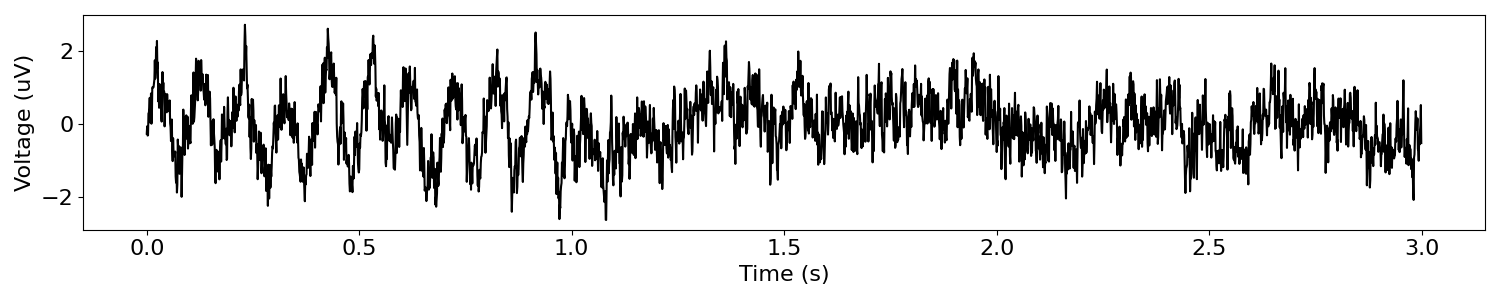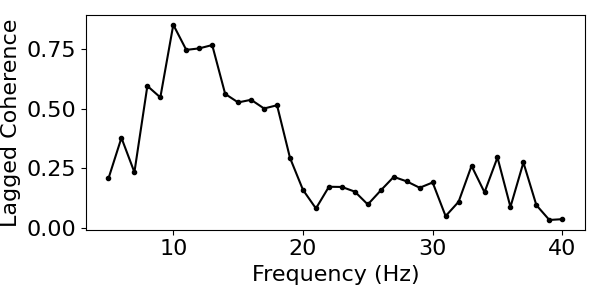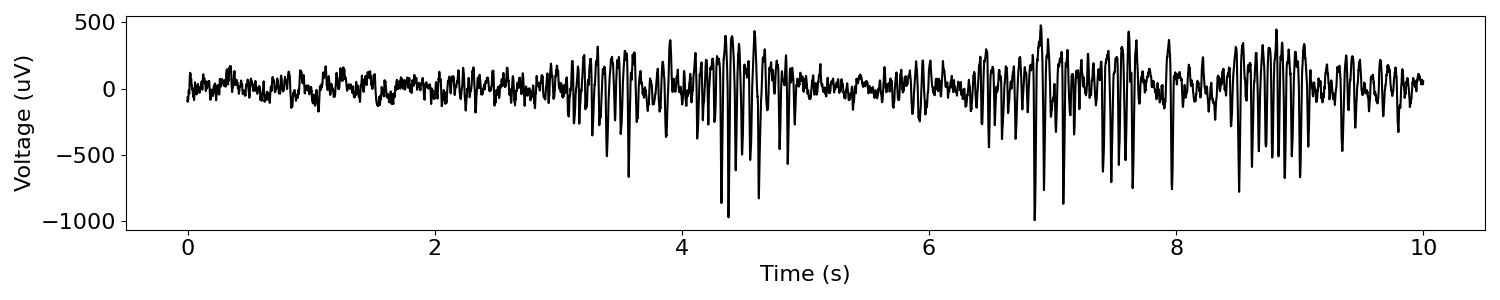# Lagged Coherence¶

Compute lagged coherence on neural signals.

This tutorial primarily covers the compute_lagged_coherence() function.

## Overview¶

Lagged coherence is a measure to quantify the rhythmicity of neural signals.

For more details on the lagged coherence measure see Fransen et al., 2015, Neuroimage.

import numpy as np

# Import the lagged coherence function
from neurodsp.rhythm import compute_lagged_coherence

# Import simulation code for creating test data
from neurodsp.sim import sim_powerlaw, sim_combined
from neurodsp.utils import set_random_seed, create_times

from neurodsp.plts.time_series import plot_time_series
from neurodsp.plts.rhythm import plot_lagged_coherence

# Set the random seed, for consistency simulating data
set_random_seed(0)


## Simulate an Example Signal¶

First, we’ll simulate an example signal that starts with a burst of alpha activity, followed by a period of only aperiodic activity.

# Set time and sampling rate
n_seconds_burst = 1
n_seconds_noise = 2
fs = 1000

# Create a times vector
times = create_times(n_seconds_burst + n_seconds_noise, fs)

# Simulate a signal component with an oscillation
components = {'sim_powerlaw' : {'exponent' : 0},
'sim_oscillation' : {'freq' : 10}}
s1 = sim_combined(n_seconds_burst, fs, components, [0.1, 1])

# Simulate a signal component with just noise
s2 = sim_powerlaw(n_seconds_noise, fs, 0, variance=0.1)

# Join signals together to approximate a 'burst'
sig = np.append(s1, s2)

# Plot example signal
plot_time_series(times, sig)## Compute lagged coherence for an alpha oscillation¶

We can compute lagged coherence with the compute_lagged_coherence() function.

# Set the frequency range to compute lagged coherence across
f_range = (8, 12)

# Compute lagged coherence
lag_coh_alpha = compute_lagged_coherence(sig, fs, f_range)

# Check the resulting value
print('Lagged coherence = ', lag_coh_alpha)


Out:

Lagged coherence =  0.853976263997615


## Compute lagged coherence across the frequency spectrum¶

Notice that lagged coherence peaks around 10Hz (the frequency of our oscillation).

However, the peak is not very specific to that frequency.

# Calculate lagged coherence across a frequency range
lag_coh_by_f, freqs = compute_lagged_coherence(sig, fs, (5, 40),
return_spectrum=True)


You can plot the lagged coherence results with plot_lagged_coherence().

# Visualize lagged coherence as a function of frequency
plot_lagged_coherence(freqs, lag_coh_by_f)## Compute lagged coherence for time segments with and without burst¶

Note that lagged coherence is greater when analyzing a neural signal that has a burst in the frequency range of interest, compared to a signal that does not have an oscillation.

# Calculate coherence for data with the burst - the 1st second of data
lag_coh_burst = compute_lagged_coherence(sig[0:fs], fs, f_range)

# Calculate coherence for data without the burst - the 2nd second of data
lag_coh_noburst = compute_lagged_coherence(sig[fs:2*fs], fs, f_range)

print('Lagged coherence, bursting = ', lag_coh_burst)
print('Lagged coherence, not bursting = ', lag_coh_noburst)


Out:

Lagged coherence, bursting =  0.9997392977993007
Lagged coherence, not bursting =  0.6340098045107515


## Compute lagged coherence of an example neural signal¶

Next, let’s apply lagged coherence to some real data.

# Download, if needed, and load example data files

# Set sampling rate, and create a times vector for plotting
fs = 1000
times = create_times(len(sig)/fs, fs)

# Plot example signal
plot_time_series(times, sig)# Set the frequency range to compute lagged coherence across
f_range = (13, 30)

# Compute lagged coherence
lag_coh_beta = compute_lagged_coherence(sig, fs, f_range)

# Check lagged coherence result
print('Lagged coherence = ', lag_coh_beta)


Out:

Lagged coherence =  0.24582055225422916


Total running time of the script: ( 0 minutes 0.190 seconds)

Gallery generated by Sphinx-Gallery